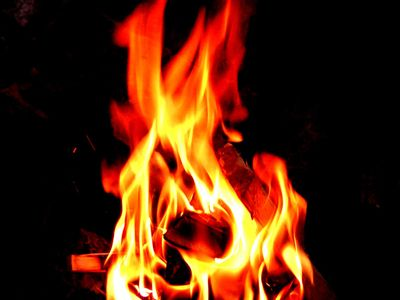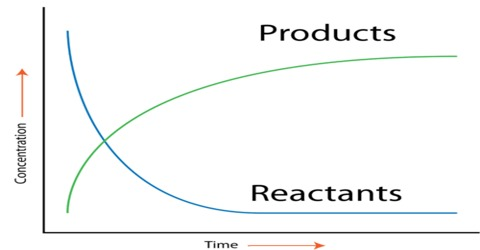# Chemical Reaction Rate Study Guide

Check out awesome, educational VR rooms on Inspirit’s mobile app (available for iOS and Android devices)🤩

## INTRODUCTION

What is the reaction rate? It is the measure of how fast a chemical reaction proceeds. Consider a combustion reaction like the burning of wood, which takes place in a few hours as compared to rusting which can take several days.Source

• In a chemical reaction, where A & B are the reactants and AB is the product or A B → AB.
• The reaction rate in chemistry is determined by how fast the concentration of A & B increases or decreases.Source

### DEFINITION OF THE RATE OF REACTION

It is defined as the estimate of the conversion in the concentration of the reactants through a certain time interval. It may be expressed as

``           Rate = Δ concentration / Δ time``

According to the above reactants A & B, the reaction rate may be written as:

``            Rate = - Δ (A) / Δ t            Rate = - Δ (B) / Δ t            Rate =   Δ (AB) / Δ t``

Here Δ(A) is the difference in the concentration of reactant A through time (t₂ – t₁). The bracket indicates the concentration in Molarity of the substance, and the negative signs show the decrease in the concentration of only the reactants.

Therefore, Δ(A) = (A₂) – (A₁) where A₂ is the final concentration and A1 is the initial concentration of reactant A. It will always be negative since the concentration of the reactant decreases with time during the reaction.

### HOW TO FIND THE RATE OF A REACTION?

A reaction rate is the conversion of the concentration of reactants with time. A 2.00M solution of A reacts with B to form AB from the above equation. It takes place in 20 seconds, and the concentration of A drops from 2.00M to 0.72M. Rate can be calculated as Δ(A) = (A₂) – (A₁) / Δ t

Therefore,

``      Rate = 0.72M – 2.00 M / 20 s              = -(-1.28) M / 20 s              = 0.064 M/s``

The reaction rate unit is measured in molarity per second or M/s. So for the above example, A’s molarity decreases at a rate of 0.064 seconds.

## CONCLUSION:

• The reaction rate in chemistry is the difference in the concentration of a reactant or product divided by the time taken.
• The rate of a reaction is measured as molarity per second.

## FAQs:

1. How do you calculate the rate of a reaction per second?

It is calculated as rate or Δ (A) = (A₂) – (A₁) / Δ t

2. How do you calculate the rate of reaction from molarity?

If the initial Molarity of a reactant was 1.00M and the final Molarity changes to 0.64M over 15 seconds, then the rate of the reaction is calculated as

Rate = 0.64M – 1.00 M / 15 s

= -(- 0.36) M / 15 s

= 0.024 M/s

The rate of the reaction is expressed as 0.024M/s.

3. What is the rate of reaction formula?

The rate may be calculated as:

rate = – Δ (A) / Δ t

We hope you enjoyed studying this lesson and learned something cool about the Chemical Reaction Rate! Join our Discord community to get any questions you may have answered and to engage with other students just like you! Don’t forget to download our App to experience our fun VR classrooms – we promise it makes studying much more fun! 😎

## SOURCES:

1. Reaction Rate. https://www.ck12.org/c/chemistry/reaction-rate/lesson/Chemical-Reaction-Rate-CHEM. Accessed 17 February 2022.
2. Reaction Rate. https://www.britannica.com/science/reaction-rate. Accessed 18 February 2022.
]]>